Test: Three Phase Bridge Inverter

# Test: Three Phase Bridge Inverter

Test Description

## 10 Questions MCQ Test Power Electronics | Test: Three Phase Bridge Inverter

Test: Three Phase Bridge Inverter for Electrical Engineering (EE) 2023 is part of Power Electronics preparation. The Test: Three Phase Bridge Inverter questions and answers have been prepared according to the Electrical Engineering (EE) exam syllabus.The Test: Three Phase Bridge Inverter MCQs are made for Electrical Engineering (EE) 2023 Exam. Find important definitions, questions, notes, meanings, examples, exercises, MCQs and online tests for Test: Three Phase Bridge Inverter below.
Solutions of Test: Three Phase Bridge Inverter questions in English are available as part of our Power Electronics for Electrical Engineering (EE) & Test: Three Phase Bridge Inverter solutions in Hindi for Power Electronics course. Download more important topics, notes, lectures and mock test series for Electrical Engineering (EE) Exam by signing up for free. Attempt Test: Three Phase Bridge Inverter | 10 questions in 30 minutes | Mock test for Electrical Engineering (EE) preparation | Free important questions MCQ to study Power Electronics for Electrical Engineering (EE) Exam | Download free PDF with solutions
 1 Crore+ students have signed up on EduRev. Have you?
Test: Three Phase Bridge Inverter - Question 1

### Identify the below given circuit.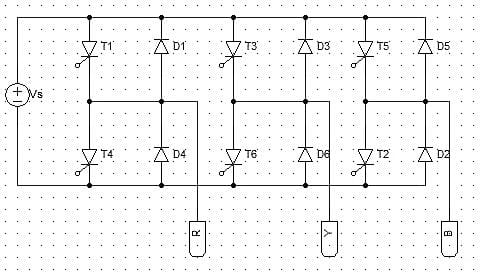Detailed Solution for Test: Three Phase Bridge Inverter - Question 1

It is a three-phase bridge type inverter. As SCRs are used as the switching device, it is called as a thyristor inverter.

Test: Three Phase Bridge Inverter - Question 2

### The below given inverter circuit is a __ step inverter.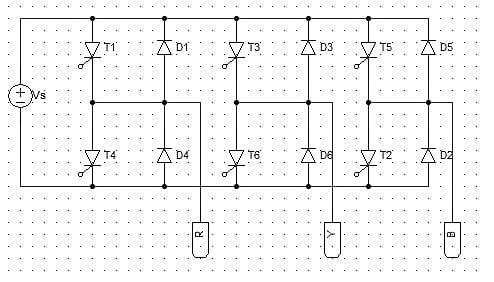Detailed Solution for Test: Three Phase Bridge Inverter - Question 2

The three-phase bridge type is a 6-step inverter. That means, the firing changes from one SCR to another 6 times per cycle.

Test: Three Phase Bridge Inverter - Question 3

### In inverters, to make the supply voltage constant

Detailed Solution for Test: Three Phase Bridge Inverter - Question 3

A large C connected across the input terminal keep the supply voltage from altering.

Test: Three Phase Bridge Inverter - Question 4

In the figure given below, for 180° mode of operation if T1 is fired at 0°. Then SCRs T3 and T5 should be fired at _________ and _________ respectively.

Detailed Solution for Test: Three Phase Bridge Inverter - Question 4

T1-T4 form the first pair. T3-T6 form the second pair, and like-wise. For the 180° mode, each SCR conducts for 180°, but the groups of SCRs lag the prior group by an angle of 120°. e.g. If T1 is fired at 0 then T3 must be fired at an angle of 0 + 120° and T5 at 120 + 120 = 240°.

Test: Three Phase Bridge Inverter - Question 5

_________ SCRs conduct from 300° to 360°.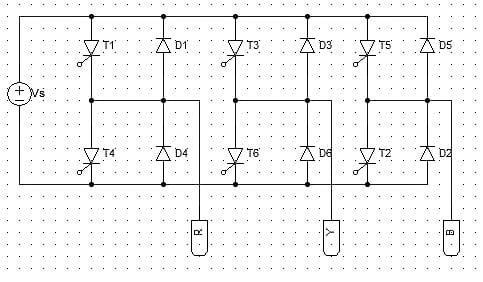Detailed Solution for Test: Three Phase Bridge Inverter - Question 5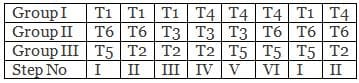Each step consists of 60°. 300° to 360° will be step VI.

Test: Three Phase Bridge Inverter - Question 6

A three-phase bridge inverter requires minimum of _______ switching devices.

Detailed Solution for Test: Three Phase Bridge Inverter - Question 6

It requires a min. 6 devices, 2 in each leg. Switching devices could be anything BJT, MOSFET or an IGBT. SCRs are used when very high power ratings are required.

Test: Three Phase Bridge Inverter - Question 7

In the three-phase bridge inverter, each step consists of

Detailed Solution for Test: Three Phase Bridge Inverter - Question 7

It is a 6 step inverter. Hence, 360/6 = 60°. This means that the SCRs are gated every 60° in proper sequence.

Test: Three Phase Bridge Inverter - Question 8

In the 180° mode VSI, ___________ devices conduct at a time.

Detailed Solution for Test: Three Phase Bridge Inverter - Question 8

Three devices conduct at a time. One from the upper pair and two from the lower pair or vice-versa.

Test: Three Phase Bridge Inverter - Question 9

For a three phase bridge inverter in the 180° mode, ___________ devices are conducting from 120° to 180°.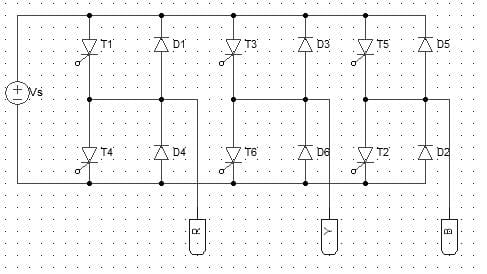Detailed Solution for Test: Three Phase Bridge Inverter - Question 9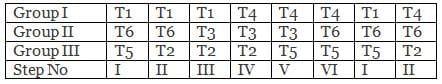Each step consists of 60°. 120° to 180° will be step III.

Test: Three Phase Bridge Inverter - Question 10

The diodes D4 and D1 will conduct from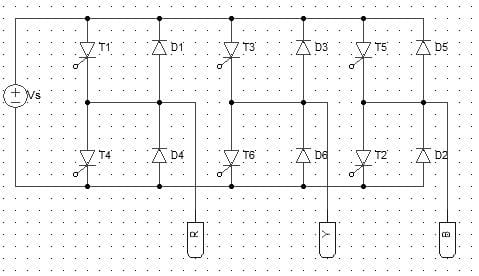Detailed Solution for Test: Three Phase Bridge Inverter - Question 10

This will depend on the nature on the load, which is not mentioned in the above problem.

## Power Electronics

5 videos|39 docs|63 tests
 Use Code STAYHOME200 and get INR 200 additional OFF Use Coupon Code
Information about Test: Three Phase Bridge Inverter Page
In this test you can find the Exam questions for Test: Three Phase Bridge Inverter solved & explained in the simplest way possible. Besides giving Questions and answers for Test: Three Phase Bridge Inverter, EduRev gives you an ample number of Online tests for practice

## Power Electronics

5 videos|39 docs|63 tests